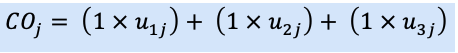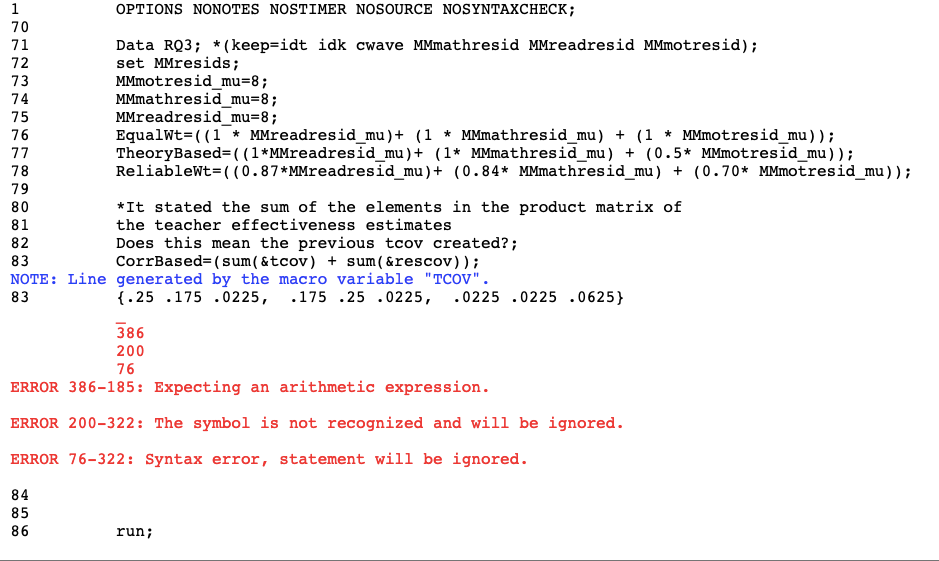## How can I fix my code for the Correlation-based as it wanted to sum the matrices?

For the research question (In terms of teacher ranking, what is the influence of constructing teacher effectiveness composites with equal weight, weight by importance, weight by reliability, and weight by residual correlation structure aggregation methods?), I have created the variables, however, I wasn’t sure about my code for the Correlation-based as it wanted to sum the matrices and I kept getting an error code. For these variables, I found the mean for each outcome and used those.

For example the equal weights variable:I created the code as follows:

EqualWt=((1 * MMreadresid_mu)+ (1 * MMmathresid_mu) + (1 * MMmotresid_mu));

Where the means of each outcome would be filled in. I got the same mean for all of the (8).

I also created the combined correlation code, but I kept getting an error as follows:I used the code highlighted in bold:

%let nt =  15; /*number of teachers*/

%let nb = 10; /*number of students*/

%let n1=3; /*number of outcomes*/

%let nanb = &nt*&nb;

/*teacher cov - this a cov matrix from the corr matrix*/

%let tcov = {.25 .175 .0225,

.175 .25 .0225,

.0225 .0225 .0625};

/*student cov*/

%let scov = {1 .2 .0225,

.2 1 .0225,

.0225 .0225 1};

/*mean vector*/

%let mv= {0,0,0};

proc iml;

gmv={50 50 30}; /*grand mean vector*/

ynew=j(&nanb,&n1,0); /*this is for the new outcomes, should be as long as the stu*tch*/

y=j(&nb,&n1,0);

tch_res=j(&nt,&n1,0);

stu_res=j(&nb,&n1,0);

call randseed(4321589);

do A = 1 to &nt;

tch_res[A,]= (RandNormal(1,&mv,&tcov)); /* tchres matrix*/

do B = 1 to &nb;

stu_res[B,]= (RandNormal(1,&mv,&scov)); /*stures matrix*/

do C = 1 to &n1;

if C = 1 then do;

y[B,1] = gmv[1,1] + tch_res[A,1] + stu_res[B,1];

end;

if C = 2 then do;

y[B,2] = gmv[1,2] + tch_res[A,1] + stu_res[B,2];

end;

if C = 3 then do;

y[B,3] = gmv[1,3] + tch_res[A,1] + stu_res[B,3];

end;

end;

end;

yy = round(y,.01);

tn = &nb*(A-1)+1;

tend = &nb*A;

ynew[(tn:tend)`,1:&n1] = yy;

y=J(&nb,&n1,0);

end;

id1 = (1:&nb)`; *print id1;

idk = repeat(id1,&nt); *print idk;

id2 = (1:&nt)`;

idt = repeat(id2,&nb); *print id21;

call sort(idt,1); *print idt;

/*save tch resids and stu resids in files*/

tchres=  tch_res;

stures=  stu_res;

create tchresids from tchres;append from tchres;close tchresids;

create sturesids from stures;append from stures;close sturesids;

ydone = idt||idk||ynew;

/*create data set to use in proc mixed*/

c = {idt idk y1 y2 y3};

create testdat from ydone [colname=c];  append from ydone;  close testdat;

quit;

/*proc print data=testdat (obs=100); run; */

data newdat;

set testdat;

y=y1; wave=1; cwave=wave; output;

y=y2; wave=2; cwave=wave; output;

y=y3; wave=3; cwave=wave; output;

keep idt idk y wave cwave;

run;

proc print data=newdat width=uniform; run;

/*run the multivariate multilevel model*/

proc mixed data=newdat covtest;

class idt idk cwave;

model y = cwave  / noint s residual outp=Resid;

random cwave / sub=idt type=un g gcorr solution;

repeated cwave / sub=idk(idt) type=un r rcorr;

ods output covparms=cp fitstatistics=fit;

run;

/*calc icc from covparm table*/

data cparms;set cp;

if subject = ' ' then subject = 'resid';

run;

proc transpose data=cp out=cp_trans;

var estimate;

id covparm subject;

run;

/* icc*/

data cp;

set cp_trans;

*****************Not correct;

iccT1 = (UN_1_1_IDT)/(UN_1_1_IDT + UN_1_1_IDK_IDT_);

iccS1 = (UN_1_1_IDK_IDT_)/(UN_1_1_IDT + UN_1_1_IDK_IDT_);

iccT2 = (UN_2_2_IDT)/(UN_2_2_IDT + UN_2_2_IDK_IDT_);

iccS2 = (UN_2_2_IDK_IDT_)/(UN_2_2_IDT + UN_2_2_IDK_IDT_);

iccT3 = (UN_3_3_IDT)/(UN_3_3_IDT + UN_3_3_IDK_IDT_);

iccS3 = (UN_3_3_IDK_IDT_)/(UN_3_3_IDT + UN_3_3_IDK_IDT_);

run;

***********;

/*data generation check*/

PROC univariate data=testdat;

var y1 y2 y3;

run;

PROC corr data=testdat cov;

var y1 y2 y3;

run;

PROC univariate data=tchresids;

var COL1 COL2 COL3;

run;

PROC univariate data=sturesids;

var COL1 COL2 COL3;

run;

/*Apply independent univariate models*/

/*Math*/

proc mixed data=testdat covtest;

class idt idk;

model y1 =  / s residual outp=mathresid;

random intercept / sub=idt type=un solution;

random intercept / sub=idk(idt) type=un solution;

ods output covparms=cpy1 fitstatistics=fity1;

run;

proc transpose data=cpy1 out=cpy1_trans;

var estimate;

id covparm subject;

run;

proc mixed data=testdat covtest ;

class idt idk;

model y2 =  / s residual outp=readresid;

random intercept / sub=idt type=vc solution;

random intercept / sub=idk(idt) type=vc solution;

ods output covparms=cpy2 fitstatistics=fity2;

run;

/*Motivation*/

proc mixed data=testdat covtest;

class idt idk;

model y3 =  / s residual outp=motresid;

random intercept / sub=idt type=vc solution;

random intercept / sub=idk(idt) type=vc solution;

ods output covparms=cpy3 fitstatistics=fity3;

run;

/RQ1/

/*Absolute bias, sum of difference between teacher level residual in tch_res from the resdiuals from MM model and the uniM models

sum of(udj - uhatdj) divided by N*/

/*change predresid file to break out residuals by outcome*/

/*predresid are from the MM model*/

run;

PROC sort data=Resid;

by cwave;

run;

Data MMresids (keep=idt idk cwave MMmathresid MMreadresid MMmotresid);

set Resid;

if cwave='1' then MMmathresid=Resid;

if cwave='3' then MMmotresid=Resid;

run;

proc sort data=MMresids;

by idt idk;

run;

set MMresids;

if cwave='2';

run;

Data MMmathresid;

set MMresids;

if cwave='1';

run;

Data MMmotresid;

set MMresids;

if cwave='3';

run;

Data MMresids1;

run;

/*create a new file with the residuals*/

Data Residuals;

Merge MMresids1 mathresid (rename=(resid=mathUtchres) keep=resid idk idt) readresid

run;

Data Bias; /*this is the new file, bias is the sum of the difference for all teachers*/

set Residuals; /*this is the file with the data*/

Mathdif= abs(MMmathresid - mathUtchres);

Motdif = abs(MMmotresid - motUtchres);

run;

proc summary Data=Bias;

output out=Biassum sum=;

output out=Bias1 mean=;

run;

/* AVERAGE BIAS, sum of all the bias across 1000 replications, for each udj, divided by 1000*/

Data AveBias;

set Bias1;

bias=sum(of bias1-bias1000);

averagebias=(sum_range)/1000;

run;

PROC corr data=Bias;

run;

PROC corr data=Bias;

var MMmathresid mathUtchres;

run;

PROC corr data=Bias;

var MMmotresid motUtchres;

run;

*To assess the difference between the corresponding (separate to joint modeled) residual correlation structures, (SRMR) was calculated.*

/*SRMR, assesses the correlation between MM and UniM, see page 49*/

/*percent of SRMR that are less than .05 = not significant*/

/*calculate SRMR- It needs to be fixed*/

PROC CALIS data=Bias;

METHOD=ML / standardized root mean square residual OUTRAM=SRMR;

run;

/* Research Q 2*/

2) Does the use of univariate or multivariate models result in different levels of model fit? ;

*UM -2LL (deviance): simplest form no other datat provided;

proc phreg data=testdat;

class idt idk;

model y1 = ;

run;

proc phreg data=testdat;

class idt idk;

model y2 = idt idk ;

run;

proc phreg data=testdat;

class idt idk;

model y3 = ;

run;

*MM -2LL (deviance): simplest form no other data provided;

proc phreg data=newdat;

class idt idk cwave;

model y= ;

run;

/* LRT, likelihood ratio test, compares differences between deviances of the models to a chi-square distr.The univariate models were considered to be nested within the multivariate model.*/

/*A significant likelihood ratio test indicates if the multivariate model is a better fit to the data than the separate univariate models.*/

/*need to sum the deviances from the UniM models*/

/*The combined deviance (Deum) of the univariate multilevel models was compared to the deviance of the multivariate model Demm */

/*the likelihood ratio test (LRT) compared the difference between the deviances (De) of the models

to a chi-square distribution with a degrees of freedom equal to the difference in the number of parameters between models.*/

/*χ2 (dfum-dfmm )= Deum-Demm;*/

/*construct an LR test statistic by making separate runs of PROC PHREG to fit the appropriate models

and then taking the positive difference in the –2logL "with covariates" statistics given by PROC PHREG in the Fit Statistics table*/

/*abs refers to absolute value, and L1 and L2 refer to the –2logL values from PROC PHREG for the two models being compared*/

/*LRT = abs(L1–L2);*/

/*Aggregating the eff estimates*/

/*Research Q 3*/

*3) In terms of teacher ranking, what is the influence of constructing teacher effectiveness composites with equal weight, weight by importance, weight by reliability, and weight by residual correlation structure aggregation methods? ;

*Residual covariances;

%let rescov={1 .7 .18,

.7 1 .18,

.18 .18 1};

var idt;

run;

proc means Data=MMmathresid mean;

var idt;

output out=MMmathresid_mu;

run;

proc means Data=MMmotresid mean;

var idt;

output out=MMmotresid_mu;

run;

/*equal weights*/

/*reliability weights, weights are .87 for math, ,84 or reading, .7 for motivation*/

/*participatory, weights are 1 for math, 1 for read, .5 for motivation*/

/*Compare the rankings*/

/*rank order the teachers based on each aggregated estimate*/

Data RQ3; *(keep=idt idk cwave MMmathresid MMreadresid MMmotresid);

set MMresids;

EqualWt=((1 * MMreadresid_mu)+ (1 * MMmathresid_mu) + (1 * MMmotresid_mu));

TheoryBased=((1*MMreadresid_mu)+ (1* MMmathresid_mu) + (0.5* MMmotresid_mu));

ReliableWt=((0.87*MMreadresid_mu)+ (0.84* MMmathresid_mu) + (0.70* MMmotresid_mu));

/*corr structure, multiply the the vector of teacher resisduals by the covariance matrix of the residuals*/

*It stated the sum of the elements in the product matrix of

the teacher effectiveness estimates

Does this mean the previous tcov created?;

CorrBased= ((sum(.25, .175, .0225, .175, .25, .0225, .0225, .0225, .0625)) * sum(1, .7, .18, .7, 1, .18, .18, .18, 1));

run;

proc print data=rq3; run;

proc sort data=RQ3;

by idt;

run;

proc corr data=RQ3 pearson spearman;

var idt;

run;

/*spearman correlation for each ordered paired set of estimates*/

/*pearson correlation for each ordered paired set of estimates*/

/*quintiles, then count teachers that move quintiles from one estimate to another*/